Computes statistics of a 2-dimensional matrix using broom::augment.htest.

stat_cross(
mapping = NULL,
data = NULL,
geom = "point",
position = "identity",
...,
na.rm = TRUE,
show.legend = NA,
inherit.aes = TRUE,
keep.zero.cells = FALSE
)

## Arguments

mapping

Set of aesthetic mappings created by aes(). If specified and inherit.aes = TRUE (the default), it is combined with the default mapping at the top level of the plot. You must supply mapping if there is no plot mapping.

data

The data to be displayed in this layer. There are three options:

If NULL, the default, the data is inherited from the plot data as specified in the call to ggplot().

A data.frame, or other object, will override the plot data. All objects will be fortified to produce a data frame. See fortify() for which variables will be created.

A function will be called with a single argument, the plot data. The return value must be a data.frame, and will be used as the layer data. A function can be created from a formula (e.g. ~ head(.x, 10)).

geom

Override the default connection with ggplot2::geom_point().

position

Position adjustment, either as a string naming the adjustment (e.g. "jitter" to use position_jitter), or the result of a call to a position adjustment function. Use the latter if you need to change the settings of the adjustment.

...

Other arguments passed on to layer(). These are often aesthetics, used to set an aesthetic to a fixed value, like colour = "red" or size = 3. They may also be parameters to the paired geom/stat.

na.rm

If TRUE, the default, missing values are removed with a warning. If TRUE, missing values are silently removed.

show.legend

logical. Should this layer be included in the legends? NA, the default, includes if any aesthetics are mapped. FALSE never includes, and TRUE always includes. It can also be a named logical vector to finely select the aesthetics to display.

inherit.aes

If FALSE, overrides the default aesthetics, rather than combining with them. This is most useful for helper functions that define both data and aesthetics and shouldn't inherit behaviour from the default plot specification, e.g. borders().

keep.zero.cells

If TRUE, cells with no observations are kept.

## Value

A ggplot2 plot with the added statistic.

## Aesthetics

stat_cross() requires the x and the y aesthetics.

## Computed variables

observed

number of observations in x,y

prop

proportion of total

row.prop

row proportion

col.prop

column proportion

expected

expected count under the null hypothesis

resid

Pearson's residual

std.resid

standardized residual

row.observed

total number of observations within row

col.observed

total number of observations within column

total.observed

total number of observations within the table

phi

phi coefficients, see augment_chisq_add_phi()

vignette("stat_cross")

## Examples

library(ggplot2)
d <- as.data.frame(Titanic)

# plot number of observations
ggplot(d) +
aes(x = Class, y = Survived, weight = Freq, size = after_stat(observed)) +
stat_cross() +
scale_size_area(max_size = 20)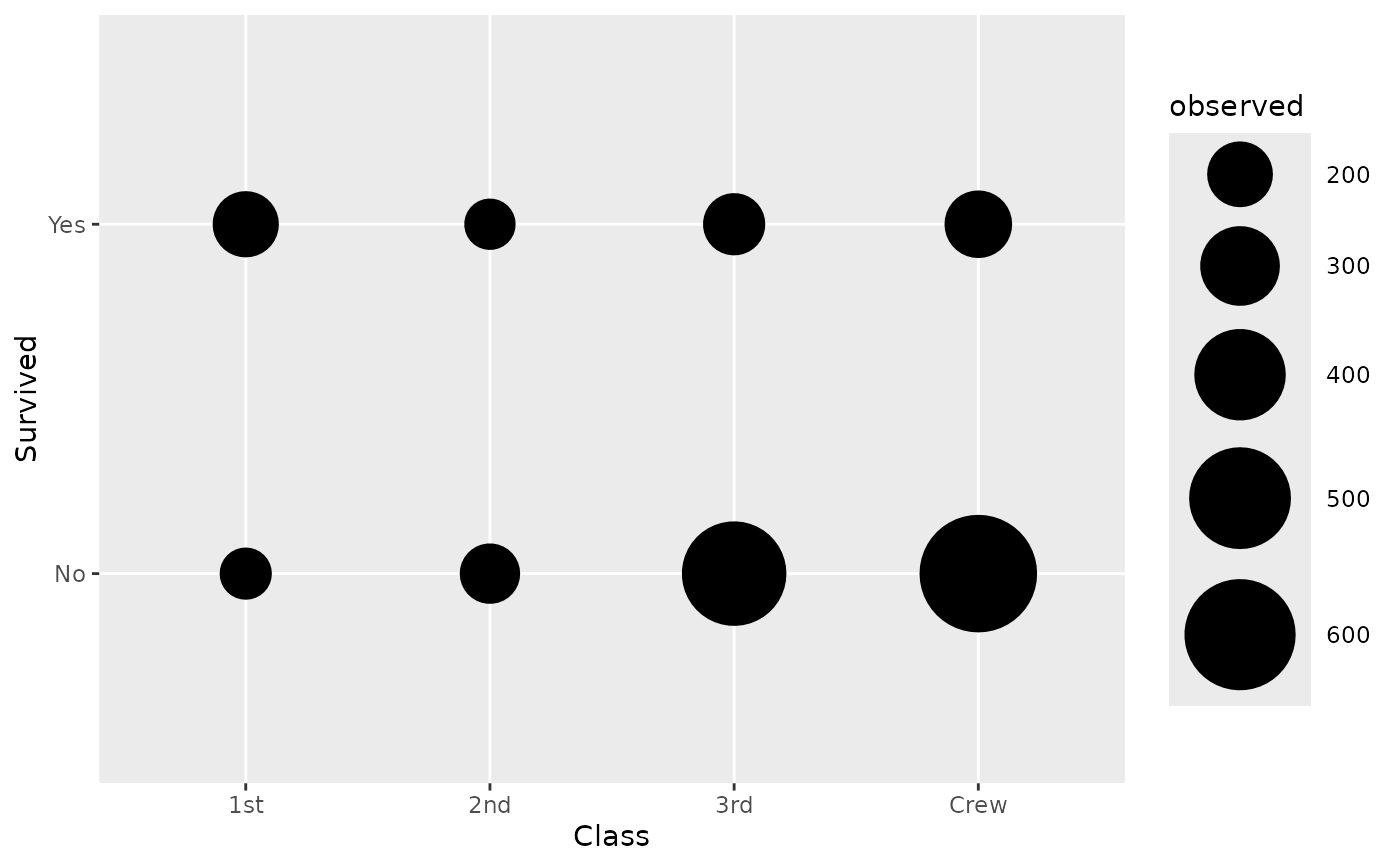# custom shape and fill colour based on chi-squared residuals
ggplot(d) +
aes(
x = Class, y = Survived, weight = Freq,
size = after_stat(observed), fill = after_stat(std.resid)
) +
stat_cross(shape = 22) +
scale_fill_steps2(breaks = c(-3, -2, 2, 3), show.limits = TRUE) +
scale_size_area(max_size = 20)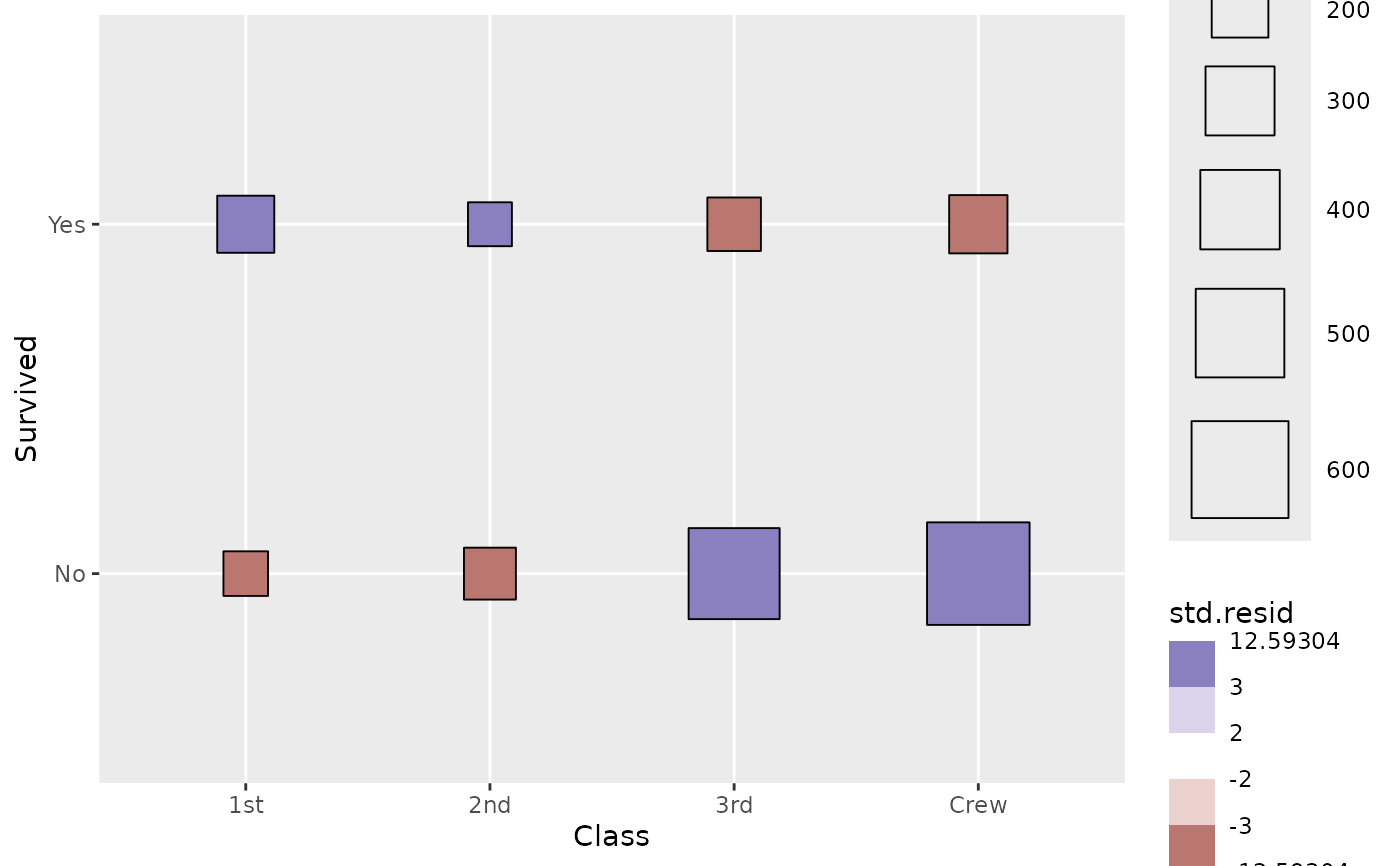# \donttest{
# custom shape and fill colour based on phi coeffients
ggplot(d) +
aes(
x = Class, y = Survived, weight = Freq,
size = after_stat(observed), fill = after_stat(phi)
) +
stat_cross(shape = 22) +
scale_fill_steps2(show.limits = TRUE) +
scale_size_area(max_size = 20)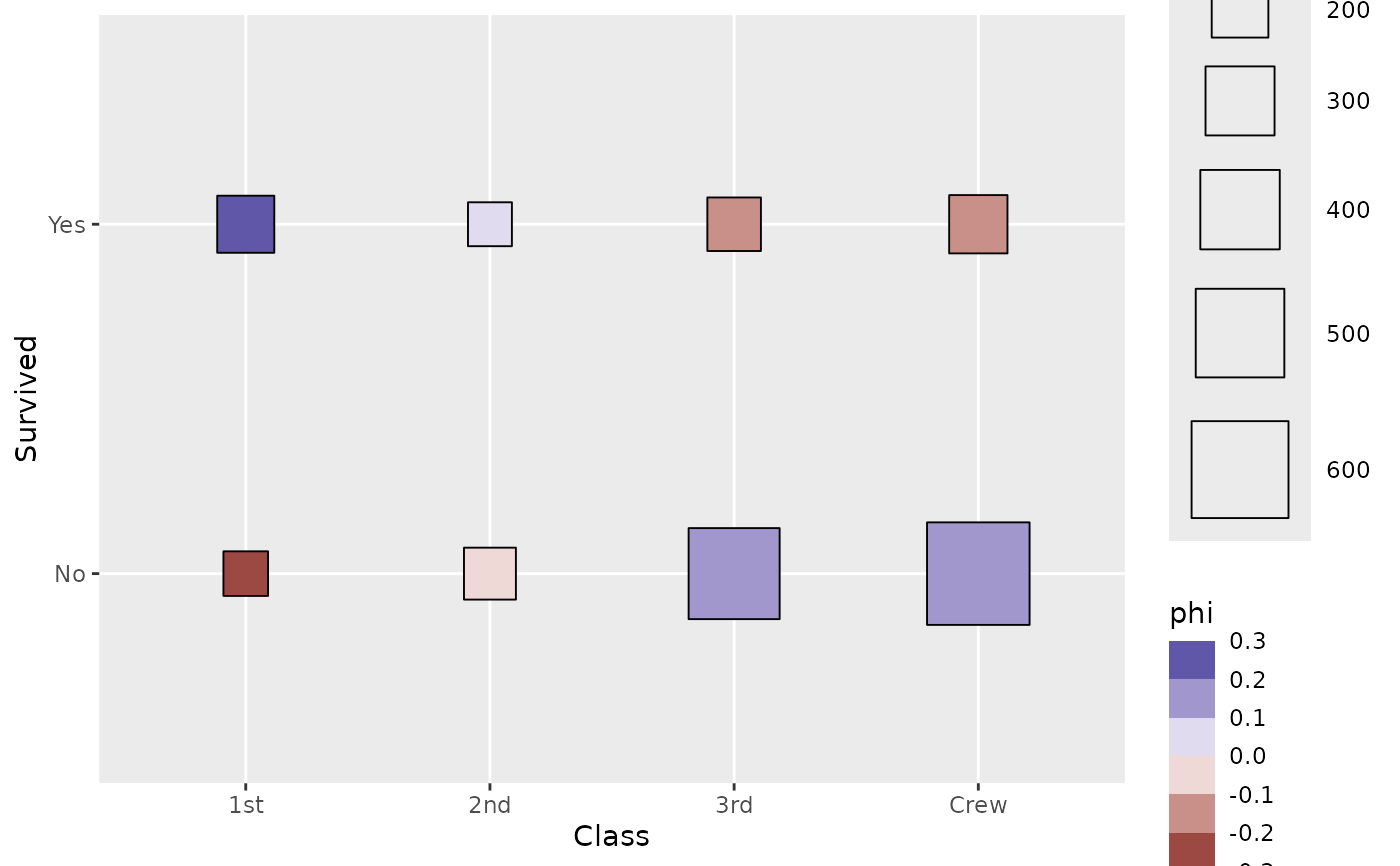# plotting the number of observations as a table
ggplot(d) +
aes(
x = Class, y = Survived, weight = Freq, label = after_stat(observed)
) +
geom_text(stat = "cross")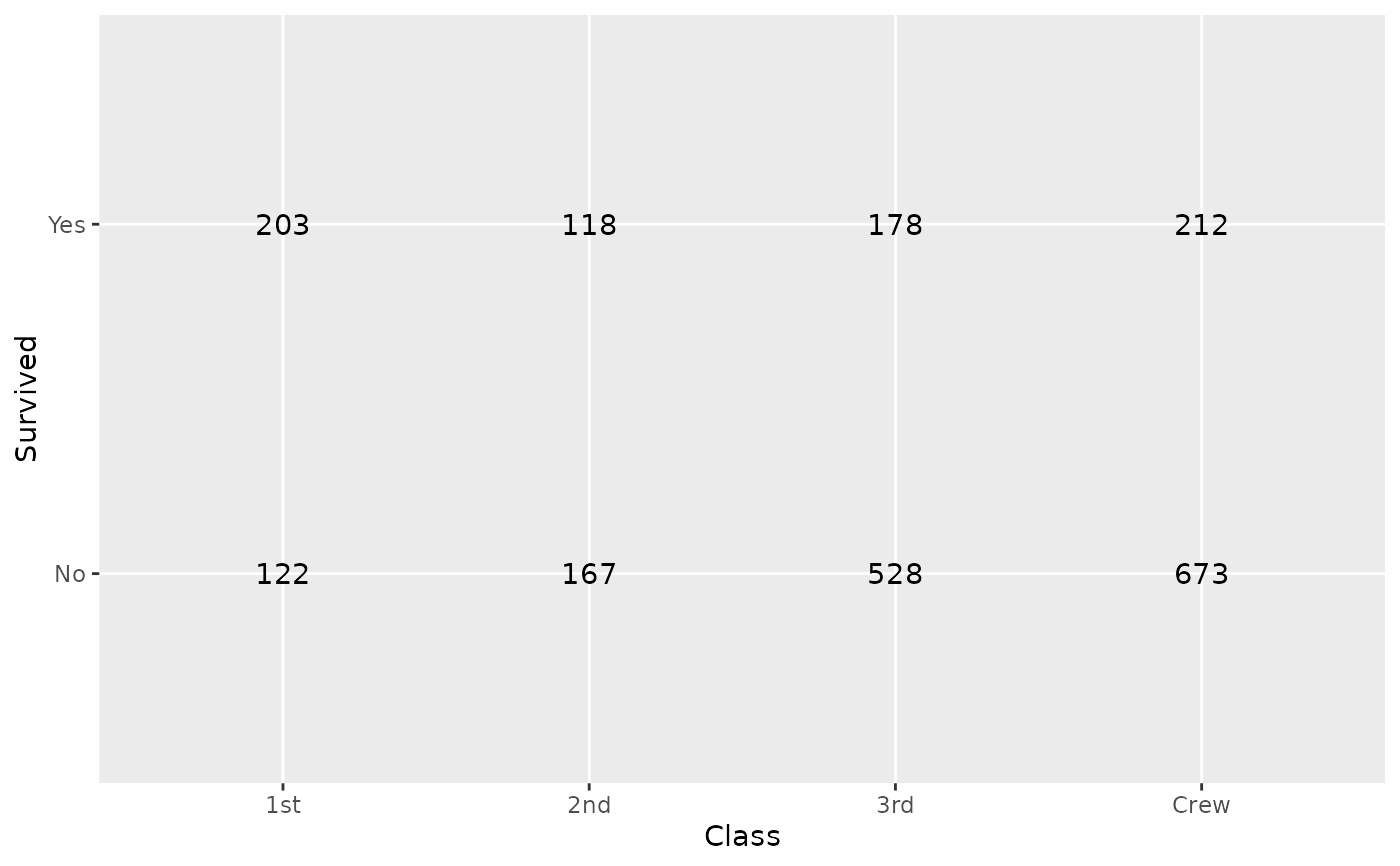# Row proportions with standardized residuals
ggplot(d) +
aes(
x = Class, y = Survived, weight = Freq,
label = scales::percent(after_stat(row.prop)),
size = NULL, fill = after_stat(std.resid)
) +
stat_cross(shape = 22, size = 30) +
geom_text(stat = "cross") +
scale_fill_steps2(breaks = c(-3, -2, 2, 3), show.limits = TRUE) +
facet_grid(Sex ~ .) +
labs(fill = "Standardized residuals") +
theme_minimal()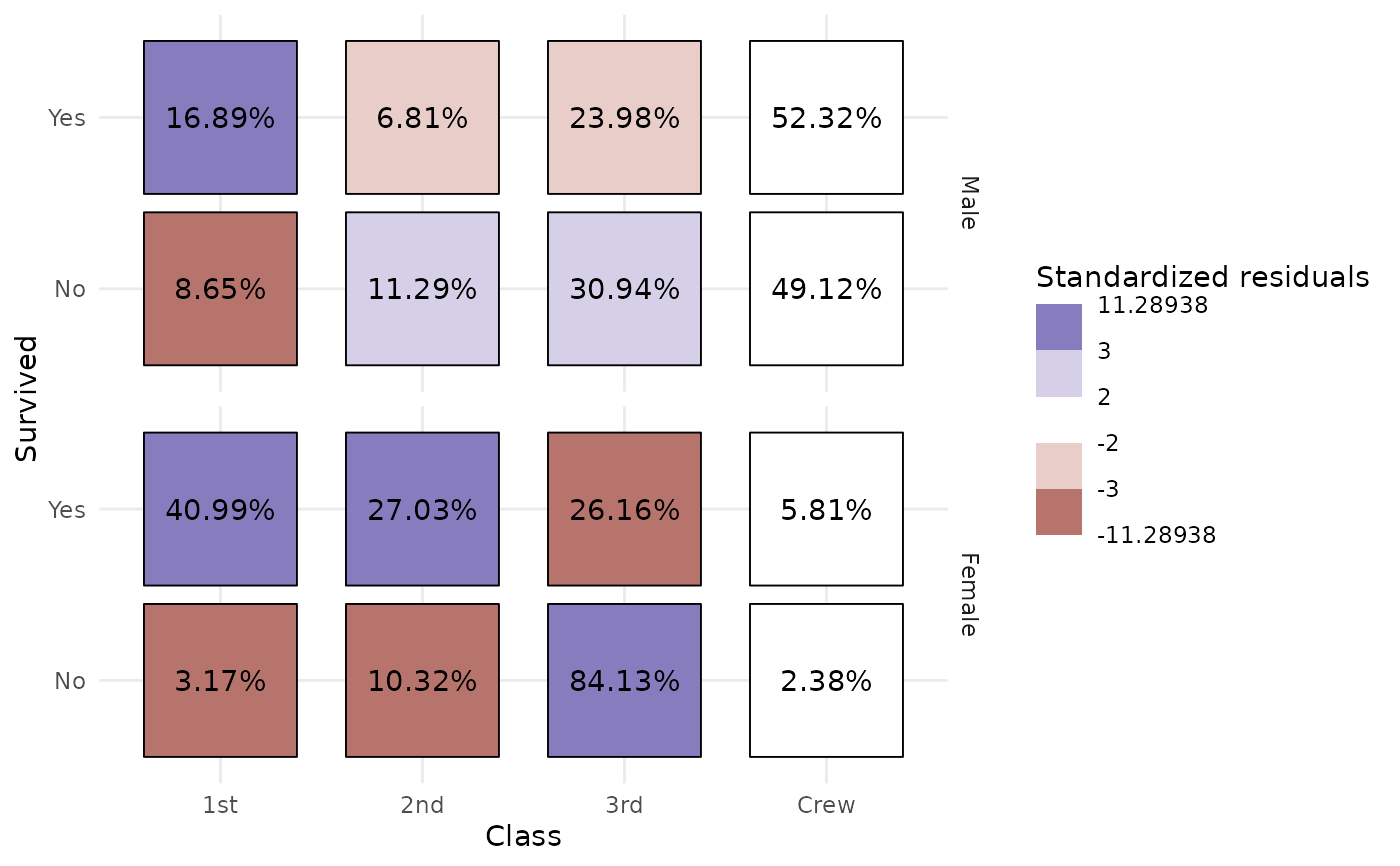# }